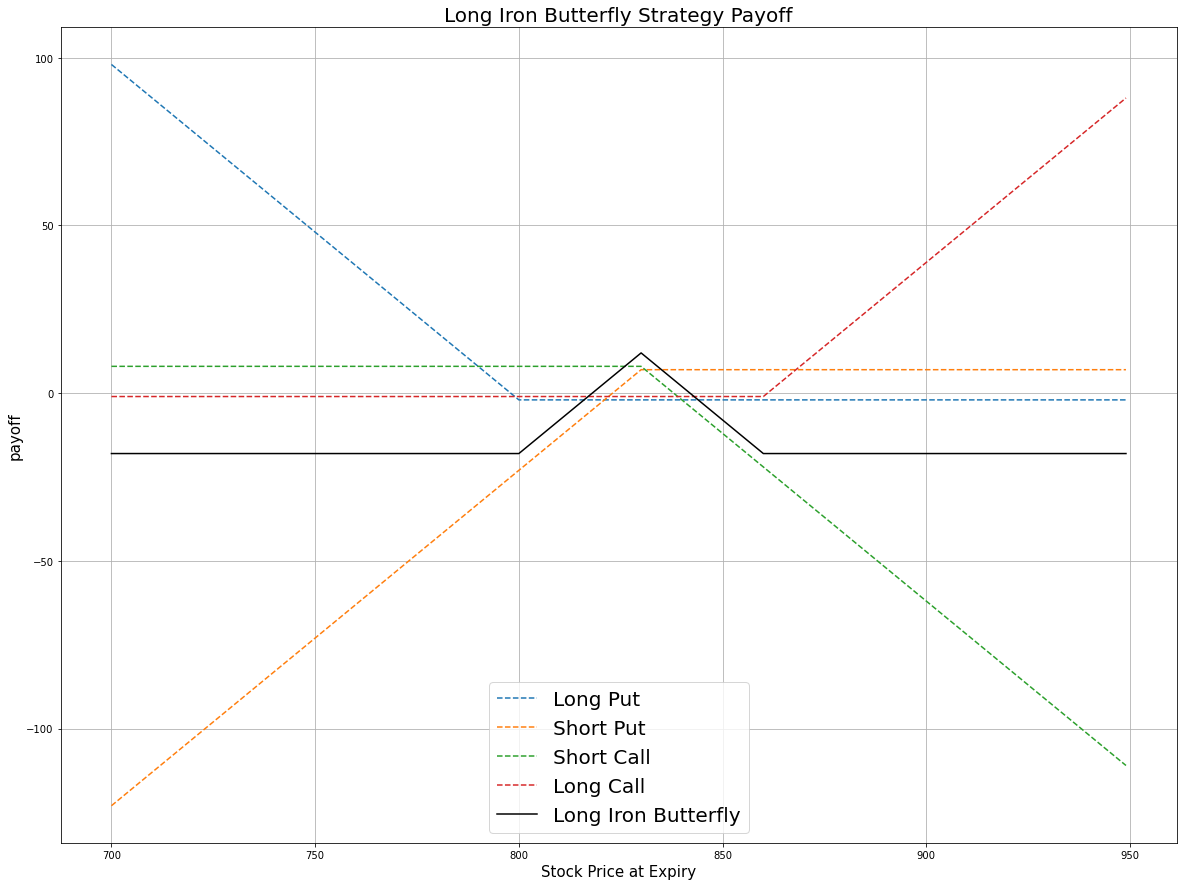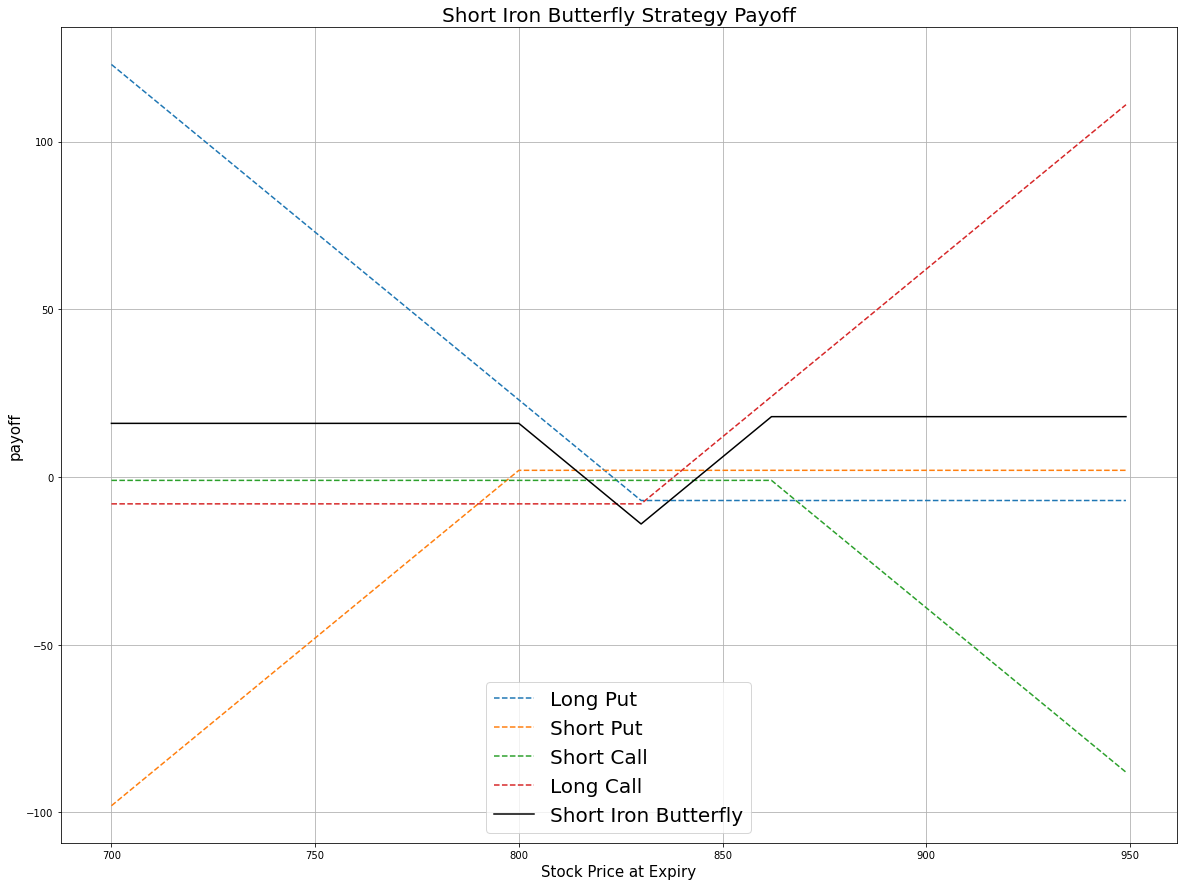# Option Strategies

## Iron Butterfly

### Introduction

Warning: There is currently no OptionStrategies method for Iron Butterfly orders, so this tutorial manually orders the individual legs in the strategy. If you manually place multi-leg orders one at a time while there is no liquidity at a strike price, you can get stuck in an unhedged position.

The Iron Butterfly is an option strategy which involves four Option contracts. All the contracts have the same underlying stock and expiration, but the order of strike prices for the four contracts is $A>B>C$. The following table describes the strike price of each contract:

PositionStrike
1 OTM call $A$
1 ATM call $B$
1 ATM put $B$
1 OTM put $C=B-(A-B)$

The iron butterfly can be long or short.

#### Long Iron Butterfly

The long iron butterfly consists of selling an OTM call, selling an OTM put, buying an ATM call, and buying an ATM put. This strategy profits from a decrease in price movement (implied volatility).

#### Short Call Butterfly

The short call butterfly consists of buying an OTM call, buying an OTM put, selling an ATM call, and selling an ATM put. This strategy profits from an increase in price movement (implied volatility) and from time decay value since ATM options decay sharper.

### Implementation

Follow these steps to implement the short iron butterfly strategy:

1. In the Initialize method, set the start date, end date, cash, and Option universe.
2. private Symbol _symbol;

public override void Initialize()
{
SetStartDate(2017, 4, 1);
SetEndDate(2017, 5, 10);
SetCash(100000);

_symbol = option.Symbol;
option.SetFilter(universe => universe.Strikes(-10, 10)
.Expiration(TimeSpan.FromDays(0), TimeSpan.FromDays(30)));
}
def Initialize(self) -> None:
self.SetStartDate(2017, 4, 1)
self.SetEndDate(2017, 5, 10)
self.SetCash(100000)

self.symbol = option.Symbol
option.SetFilter(-10, 10, timedelta(0), timedelta(30))
3. In the OnData method, select the contracts in the strategy legs.
4. public override void OnData(Slice slice)
{
if (Portfolio.Invested) return;

// Get the OptionChain
var chain = slice.OptionChains.get(_symbol, null);
if (chain == null || chain.Count() == 0) return;

// Separate the call and put contracts
var calls = chain.Where(x => x.Right == OptionRight.Call);
var puts = chain.Where(x => x.Right == OptionRight.Put);
if (calls.Count() == 0 || puts.Count() == 0) return;

// Get the ATM strike price
var atmStrike = chain.OrderBy(x => Math.Abs(x.Strike - chain.Underlying.Price)).First().Strike;

// Select the OTM contracts
var otmCallContract = calls.OrderBy(x => x.Strike).Last();
var otmPutContract = puts.OrderBy(x => x.Strike).First();

// Select the ATM contracts
var atmCallContract = calls.Where(x => x.Strike == atmStrike).First();
var atmPutsContract = puts.Where(x => x.Strike == atmStrike).First();
def OnData(self, slice: Slice) -> None:
if self.Portfolio.Invested: return

# Get the OptionChain
chain = slice.OptionChains.get(self.symbol, None)
if not chain: return

# Separate the call and put contracts
call = [i for i in chain if i.Right == 0]
put = [i for i in chain if i.Right == 1]
if len(call) == 0 or len(put) == 0 : return

# Select the OTM contracts
call_contracts = sorted(call, key = lambda x: x.Strike)
put_contracts = sorted(put, key = lambda x: x.Strike)
otm_call = call_contracts[-1]
otm_put = put_contracts

# Select the ATM contracts
atm_put = sorted(put_contracts, key = lambda x: abs(chain.Underlying.Price - x.Strike))
atm_call = sorted(call_contracts, key = lambda x: abs(chain.Underlying.Price - x.Strike))

5. In the OnData method, submit the orders.
6. Sell(atmPutsContract.Symbol, 1);
Sell(atmCallContract.Symbol, 1);
Buy(otmPutContract.Symbol, 1);
self.Sell(atm_put.Symbol, 1)
self.Sell(atm_call.Symbol, 1)
self.Buy(otm_put.Symbol, 1)

### Strategy Payoff

The iron butterfly can be long or short.

#### Long Iron Butterfly

The long iron butterfly is a limited-reward-limited-risk strategy. The payoff is

$$\begin{array}{rcll} C^{OTM}_T & = & (S_T - K^C_{OTM})^{+}\\ C^{ATM}_T & = & (S_T - K^C_{ATM})^{+}\\ P^{OTM}_T & = & (K^P_{OTM} - S_T)^{+}\\ P^{ATM}_T & = & (K^P_{ATM} - S_T)^{+}\\ P_T & = & (C^{ATM}_T + P^{ATM}_T - C^{OTM}_T - P^{OTM}_T - C^{ATM}_0 - P^{ATM}_0 + C^{OTM}_0 + P^{OTM}_0)\times m - fee \end{array}$$ $$\begin{array}{rcll} \textrm{where} & C^{OTM}_T & = & \textrm{OTM call value at time T}\\ & C^{ATM}_T & = & \textrm{ATM call value at time T}\\ & P^{OTM}_T & = & \textrm{OTM put value at time T}\\ & P^{ATM}_T & = & \textrm{ATM put value at time T}\\ & S_T & = & \textrm{Underlying asset price at time T}\\ & K^C_{OTM} & = & \textrm{OTM call strike price}\\ & K^C_{ATM} & = & \textrm{ATM call strike price}\\ & K^P_{OTM} & = & \textrm{OTM put strike price}\\ & K^P_{ATM} & = & \textrm{ATM put strike price}\\ & P_T & = & \textrm{Payout total at time T}\\ & C^{OTM}_0 & = & \textrm{OTM call value at position opening (credit received)}\\ & C^{ATM}_0 & = & \textrm{ATM call value at position opening (debit paid)}\\ & P^{OTM}_0 & = & \textrm{OTM put value at position opening (credit received)}\\ & P^{ATM}_0 & = & \textrm{ATM put value at position opening (debit paid)}\\ & m & = & \textrm{Contract multiplier}\\ & T & = & \textrm{Time of expiration} \end{array}$$

The following chart shows the payoff at expiration:The maximum profit is $K^C_{OTM} - K^C_{ATM} - C^{ATM}_0 - P^{ATM}_0 + C^{OTM}_0 + P^{OTM}_0$. It occurs when the underlying price is below the OTM put strike price or above the OTM call strike price at expiration.

The maximum loss is the net debit paid, $C^{OTM}_0 + P^{OTM}_0 - C^{ATM}_0 - P^{ATM}_0$. It occurs when the underlying price stays the same as when you opened the trade.

If the Option is American Option, there is a risk of early assignment on the sold contracts.

#### Short Call Butterfly

The short call butterfly is a limited-reward-limited-risk strategy. The payoff is

$$\begin{array}{rcll} C^{OTM}_T & = & (S_T - K^C_{OTM})^{+}\\ C^{ATM}_T & = & (S_T - K^C_{ATM})^{+}\\ P^{OTM}_T & = & (K^P_{OTM} - S_T)^{+}\\ P^{ATM}_T & = & (K^P_{ATM} - S_T)^{+}\\ P_T & = & (C^{OTM}_T + P^{OTM}_T - C^{ATM}_T - P^{ATM}_T - C^{OTM}_0 - P^{OTM}_0 + C^{ATM}_0 + P^{ATM}_0)\times m - fee \end{array}$$ $$\begin{array}{rcll} \textrm{where} & C^{OTM}_T & = & \textrm{OTM call value at time T}\\ & C^{ATM}_T & = & \textrm{ATM call value at time T}\\ & P^{OTM}_T & = & \textrm{OTM put value at time T}\\ & P^{ATM}_T & = & \textrm{ATM put value at time T}\\ & S_T & = & \textrm{Underlying asset price at time T}\\ & K^C_{OTM} & = & \textrm{OTM call strike price}\\ & K^C_{ATM} & = & \textrm{ATM call strike price}\\ & K^P_{OTM} & = & \textrm{OTM put strike price}\\ & K^P_{ATM} & = & \textrm{ATM put strike price}\\ & P_T & = & \textrm{Payout total at time T}\\ & C^{OTM}_0 & = & \textrm{OTM call value at position opening (debit paid)}\\ & C^{ATM}_0 & = & \textrm{ATM call value at position opening (credit received)}\\ & P^{OTM}_0 & = & \textrm{OTM put value at position opening (debit paid)}\\ & P^{ATM}_0 & = & \textrm{ATM put value at position opening (credit received)}\\ & m & = & \textrm{Contract multiplier}\\ & T & = & \textrm{Time of expiration} \end{array}$$

The following chart shows the payoff at expiration:The maximum profit is the net credit received, $C^{ATM}_0 + P^{ATM}_0 - C^{OTM}_0 - P^{OTM}_0$. It occurs when the underlying price stays the same as when you opened the trade.

The maximum loss is $K^C_{OTM} - K^C_{ATM} - C^{ATM}_0 - P^{ATM}_0 + C^{OTM}_0 + P^{OTM}_0$. It occurs when the underlying price is below the OTM put strike price or above the OTM call strike price at expiration.

If the Option is American Option, there is a risk of early assignment on the sold contracts.

### Example

The following table shows the price details of the assets in the algorithm:

AssetPrice ($)Strike ($)
OTM call1.85857.50
OTM put2.75810.00
ATM call8.10832.00
ATM put7.40832.00
Underlying Equity at expiration851.20-

Therefore, the payoff is

$$\begin{array}{rcll} C^{OTM}_T & = & (S_T - K^C_{OTM})^{+}\\ & = & (851.20-857.50)^{+}\\ & = & 0\\ C^{ATM}_T & = & (S_T - K^C_{ATM})^{+}\\ & = & (851.20-832.00)^{+}\\ & = & 19.20\\ P^{OTM}_T & = & (K^P_{OTM} - S_T)^{+}\\ & = & (832.00-851.20)^{+}\\ & = & 0\\ P^{ATM}_T & = & (K^P_{ATM} - S_T)^{+}\\ & = & (810.00-851.20)^{+}\\ & = & 0\\ P_T & = & (C^{OTM}_T + P^{OTM}_T - C^{ATM}_T - P^{ATM}_T - C^{OTM}_0 - P^{OTM}_0 + C^{ATM}_0 + P^{ATM}_0)\times m - fee\\ & = & (0+0-19.20-0+8.10+7.40-1.85-2.75)\times100-1\times4\\ & = & -834 \end{array}$$

So, the strategy losses \$834.

The following algorithm implements a short iron butterfly Option strategy:

You can also see our Videos. You can also get in touch with us via Discord.